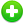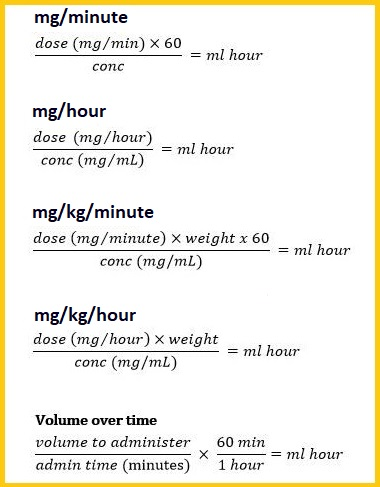#Dosage Calculator# Conversion Tool

Please clickin the following header, to convert the other units.

## Medication (Universal Formula) Calculation :

In the universal formula, the desired dose (D) is the dose prescribed by the provider. The amount on hand (H) is the dose on the container label. The Quantity (Q) is the form and amount in which the drug is supplied (i.e. tablet, capsule, liquid).

This is traditional formula and dimensional analysis. Nurses can practice to become proficient in that method.To use this formula, divide the desired amount by the amount on hand and multiply by the volume or quantity.

X = DH × Q

Where,

D = Desired Dose

Q = Quantity of Solution

H = Strength on Hand or Amount on hand

X = Unknown quantity of Drug

Example1 :

Physician orders 500 mg of ibuprofen (desired Dose) for a patient and you have 250 mg (Quantity on Hand) tablets (Quantity of solution) on hand.

Solution: X = ( D ÷ H ) × Q

500mg ÷ 250 mg × 1 tablet = 2 tablets

Example2 :

Physician orders 1500 mg of liquid ibuprofen for a patient. Quantity of Ibuprofen is 500 mg in 1 cc, how much will you administer

Solution: 1500 mg ÷ 500 mg × 1cc = 3 cc

Example3 :

Administer digoxin 0.5 mg IV daily. The drug concentration available from the pharmacy is digoxin 0.25 mg/mL. How many mL will you need to administer a 0.5 mg dose

Solution: 0.5 mg ÷ 0.25 mg × 1 = 2 mL

Quick reference: Universal formulas :

These formulas will provide a simple and concise method for accurate computation using basic calculations.###Recommended Pages ►

Sleep Duration Time Chart

Snellen Eye Chart

Fever Temperature Chart

Hemoglobin Level Chart

Height Weight Chart

mmol/L to mg/dl Blood Glucose Chart